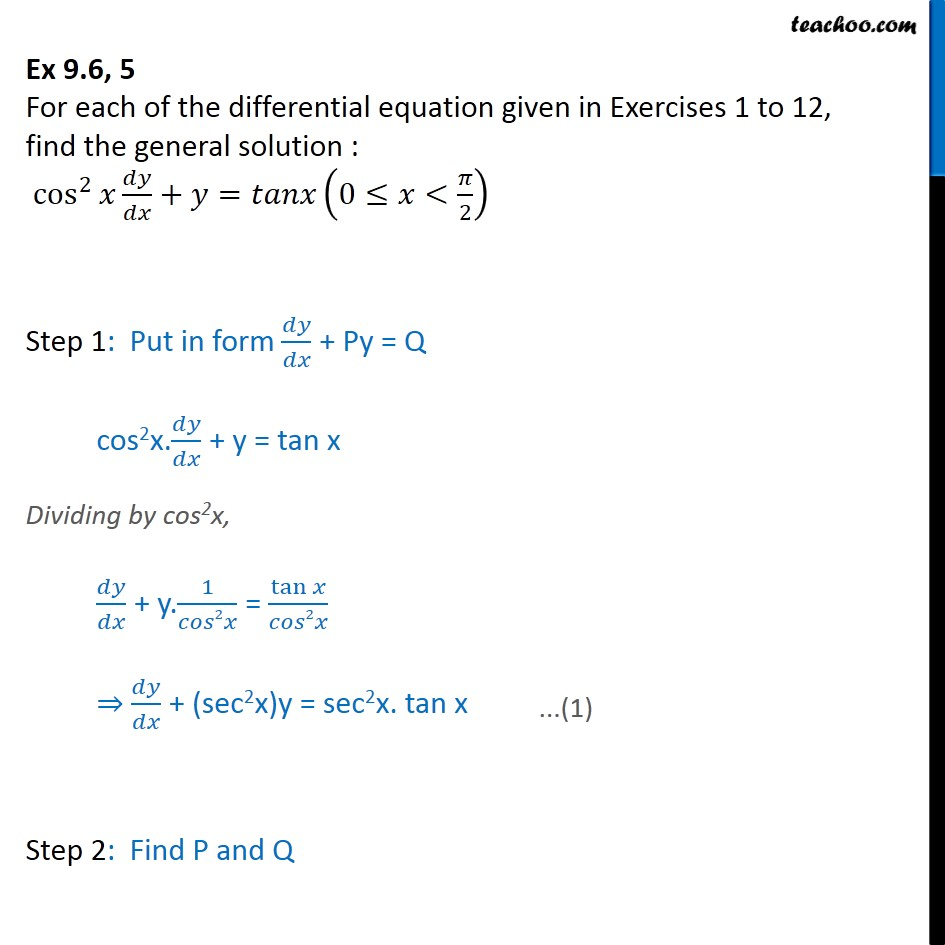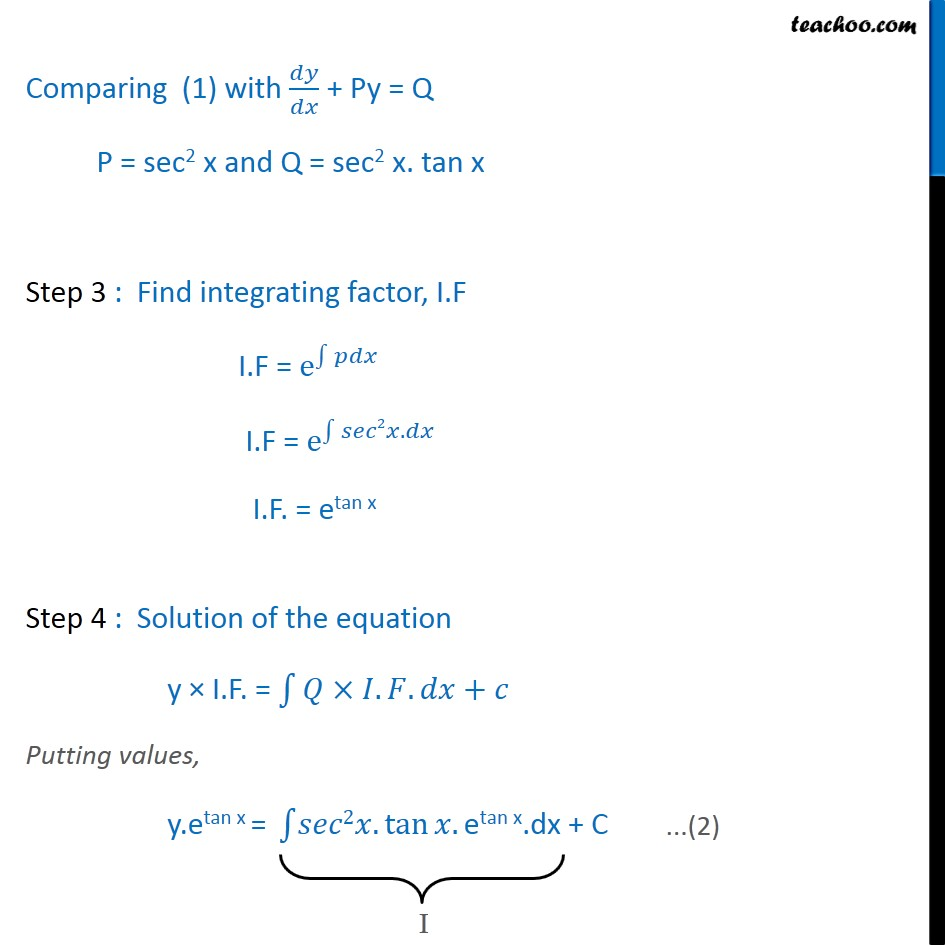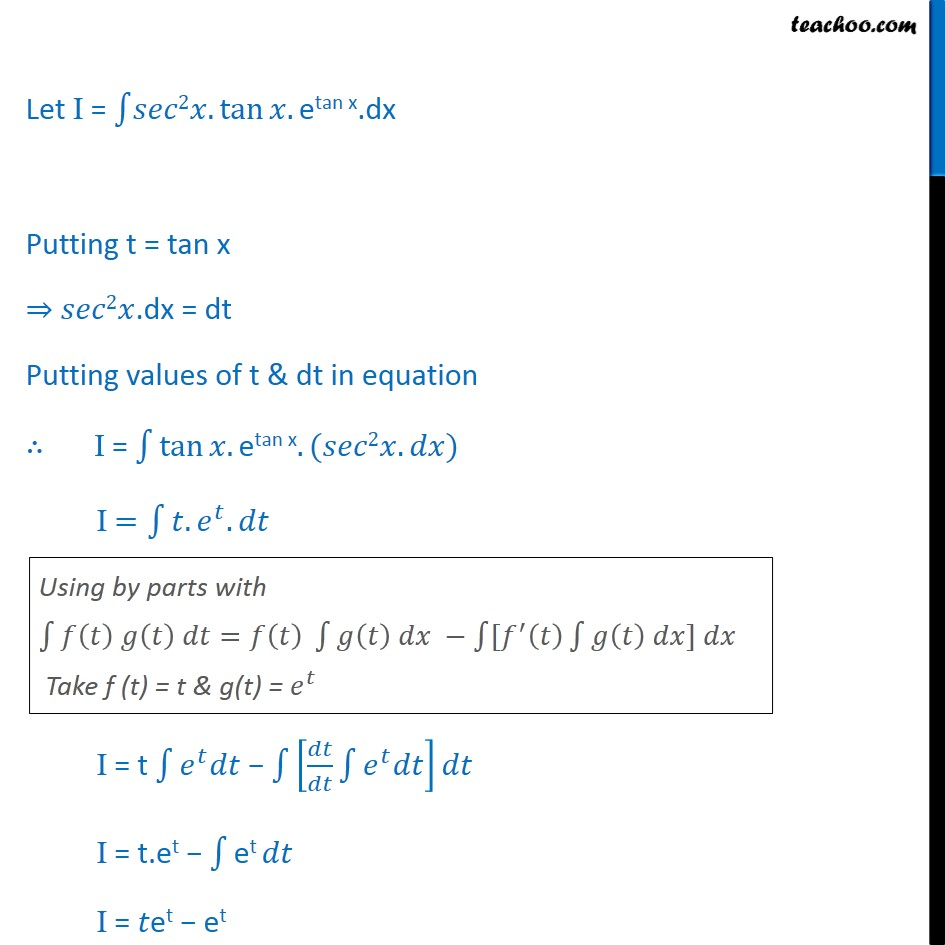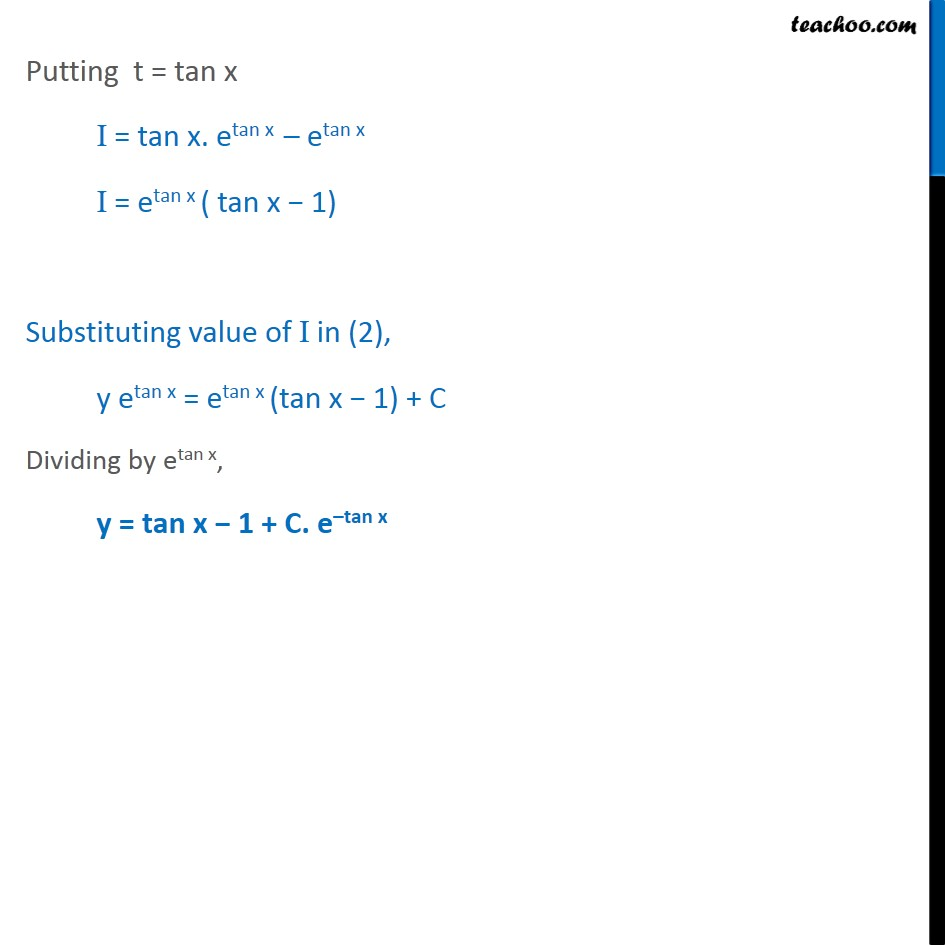Ex 9.6

Chapter 9 Class 12 Differential Equations
Serial order wiseGet live Maths 1-on-1 Classs - Class 6 to 12

### Transcript

Ex 9.6, 5 For each of the differential equation given in Exercises 1 to 12, find the general solution : cos﷮2﷯﷮𝑥 𝑑𝑦﷮𝑑𝑥﷯+𝑦=𝑡𝑎𝑛𝑥 0≤𝑥< 𝜋﷮2﷯﷯﷯ Step 1: Put in form 𝑑𝑦﷮𝑑𝑥﷯ + Py = Q cos2x. 𝑑𝑦﷮𝑑𝑥﷯ + y = tan x Dividing by cos2x, 𝑑𝑦﷮𝑑𝑥﷯ + y. 1﷮𝑐𝑜𝑠2𝑥﷯ = tan﷮𝑥﷯﷮𝑐𝑜𝑠2𝑥﷯ ⇒ 𝑑𝑦﷮𝑑𝑥﷯ + (sec2x)y = sec2x. tan x Step 2: Find P and Q Comparing (1) with 𝑑𝑦﷮𝑑𝑥﷯ + Py = Q P = sec2 x and Q = sec2 x. tan x Step 3 : Find integrating factor, I.F I.F = e﷮ ﷮﷮𝑝𝑑𝑥﷯﷯ I.F = e﷮ ﷮﷮𝑠𝑒𝑐2𝑥.𝑑𝑥﷯ ﷯ I.F. = etan x Step 4 : Solution of the equation y × I.F. = ﷮﷮𝑄×𝐼.𝐹.𝑑𝑥﷯+𝑐 Putting values, y.etan x = ﷮﷮𝑠𝑒𝑐2𝑥. tan﷮𝑥.﷯﷯etan x.dx + C Let I = ﷮﷮𝑠𝑒𝑐2𝑥. tan﷮𝑥.﷯﷯etan x.dx Putting t = tan x ⇒ 𝑠𝑒𝑐2𝑥.dx = dt Putting values of t & dt in equation ∴ I = ﷮﷮ tan﷮𝑥.etan x.(𝑠𝑒𝑐2𝑥.𝑑𝑥)﷯﷯ I = ﷮﷮𝑡. 𝑒﷮𝑡﷯.𝑑𝑡﷯ I = t ﷮﷮ 𝑒﷮𝑡﷯𝑑𝑡﷯ − ﷮﷮ 𝑑𝑡﷮𝑑𝑡﷯ ﷮﷮ 𝑒﷮𝑡﷯𝑑𝑡﷯﷯﷯𝑑𝑡 I = t.et − ﷮﷮et 𝑑𝑡﷯ I = 𝑡et − et . Putting t = tan x I = tan x. etan x – etan x I = etan x ( tan x − 1) Substituting value of I in (2), y etan x = etan x (tan x − 1) + C Dividing by etan x, y = tan x − 1 + C. e–tan x